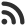Feed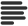Articles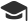Tutorials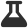CodingLab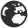CommunityLeaderboardFounder & Programmer at HackersFriend Updated Nov. 21, 2019, 7:57 p.m. ⋅ 86 views

# Add two numbers without using arithmetic operators

We want to add 2 numbers without using arithmetic operator ( + , - , / , * ) . The trick here is to use properties of XOR.

So, let's try to understand how it works.

We'll use Half adder logic here. Points to note down are:

• Sum of two bits can be obtained by performing XOR (^) of the two bits.
• Carry bit can be obtained by performing AND (&) of two bits.

The above logic can be used to add 2 single bits. Now, we are going to extend this logic for integers. Let's say we have to 2 integers, X and Y. In order to get sum of X and Y we'll first convert both of them into their binary repersentation.

Here there can be 2 cases:

• X and Y don't have any common position in which bits of both are set.
• Or they might have positions in which bits are set in both.

For the first case, we can easily get sum of X and Y by performing XOR. But for the second case, we need to use bitwise AND( & ). Bitwise AND of X and Y will give all carry bits. Bitwise AND of X and Y gives all carry bits. We will calculate (X & Y) << 1 and add it to X ^ Y to get the required result.

Here is a function of implementation of this apporach.

``````int Add(int x, int y)
{
// Loop until there is no carry left
while (y != 0)
{
// carry now contains common set bits of x and y

int carry = x & y;

// Sum of bits of x and y where at least one of the bits is not set

x = x ^ y;

// Carry is shifted by one so that adding
// it to x gives the required sum
y = carry << 1;
}

return x;
}``````

Here is Recursive version of above function.

``````int Add(int x, int y)
{
if (y == 0)
return x;
else
return Add( x ^ y, (x & y) << 1);
}
``````

Here is a complete example with Main function.

``````// C++ Program to add two numbers
// without using arithmetic operator

#include <bits/stdc++.h>
using namespace std;

{
if (y == 0)
return x;
else
return Add( x ^ y, (x & y) << 1);
}

int main()
{
return 0;
}
``````
``34``

 arrow_upward UpvoteComment arrow_downward downvote

Events

Nov. 28, 2018, 5:30 p.m.

Python from zero to hero

place Delhi

Aug. 13, 2018, 5:30 p.m.

Python from zero to hero

place Bangalore ( HackersFriend office BTM Layout)